# How to Create or Insert A Textbox Control with Scroll Bar in Excel

Sometimes we need to add a textbox into current worksheet to edit some comments for purpose. And if we want to type a long comment but with textbox length or width limit, we need to add a scroll bar for this textbox, then we can drag the scroll bar to show all contents. Adding scroll bar can prevent contents in textbox from truncating, this article will show you the method to create a textbox with scroll bar in excel.

Expectation:

Add a scrollbar for textbox control.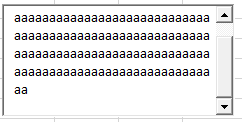## Method: Create or Insert A Textbox with Scroll Bar

Step 1: Click Developer in ribbon, then click Insert arrow button to load Form Controls. Select Text Box control.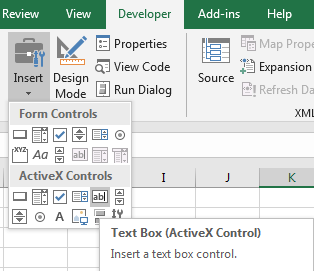Step 2: Drag your mouse to draw a textbox.Step 3: If you want to adjust the size of textbox, click Design Mode under Controls group.Then click on textbox control, then selected textbox is in design mode and adjustable, you can drag any circle to extend or reduce the size.

Step 4: After adjusting textbox size, click Properties under Developer.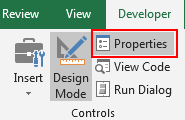And Properties window pops up.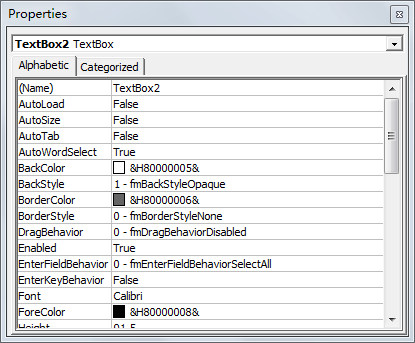Step 5: Drag the scrollbar till MultiLine and ScorllBars show on the window. Set MultiLine with True, and in ScrollBars dropdown list, select the third choice ‘2 – fmScrollBarsVertical’.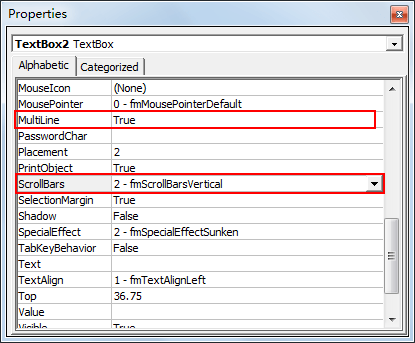Normally we often add vertical scrollbar, if you want to add horizontal scrollbar, you can select the second choice ‘1 – fmScrollBarsHorizontal’.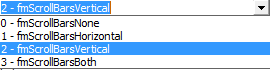Step 6: Click Design Mode again to quit design mode. Then type a long sentence into textbox.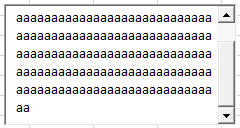When entering texts over the textbox, a scrollbar will be displayed. You can drag scrollbar to show all texts.

Related Posts

Calculate Number of Hours between Two Times

Calculating the difference between two times might be a valuable statistic for subsequent computations or averages, whether you're producing a time sheet for staff or recording personal exercises. While Excel has a plethora of complex functions, including date and time ...

Calculate Loan Interest in Given Year

When you borrow money, you are supposed to repay it gradually. Lenders, on the other hand, want to be compensated for their services and the risk they incur by lending you money. That is, you will not just repay the ...

Calculate Interest Rate for Loan

The interest rate is the fee charged by a lender to a borrower and is expressed as a percentage of the principal—the lent amount. The interest rate on a loan is often expressed as an annual percentage rate, abbreviated as ...

Calculate Interest for Given Period

Using the IPMT function in Excel, we can compute the interest payment on any loan. This step-by-step tutorial will guide Excel users of all skill levels through the process to calculate interest for given period. Finally, the formula: =IPMT(B3/12,1,B5,-B2) The ...

How To Use Excel GCD Function

This post will guide you how to use Excel GCD function with syntax and examples in Microsoft excel. Description The Excel GCD function Returns the greatest common divisor of two or more integers. So you can use the GCD function ...

Calculate A Ratio From Two Numbers In Excel

In elementary mathematics, a ratio is a connection or comparison between two or more integers. For example, ratios are often expressed as ":" to demonstrate the relationship between two numbers. You would think that manually calculating a ratio from two ...

How To Use Excel RRI Function

This post will guide you how to use Excel RRI function with syntax and examples in Microsoft excel. Description The Excel RRI function Returns an equivalent interest rate for the growth of an investment. So you can use the RRI ...

CAGR Formula Examples in Excel

CAGR in Excel is a formula that calculates the compound annual growth rate for any invested amount over the specified years or timeframe. Although there is no direct function in Excel that can help us identify the CAGR value, there ...

Build Hyperlink With VLOOKUP in Excel

You might have come across a task in which you were assigned to build hyperlinks, which seems very easy, and if you are new to excel or don't have enough experience with it, then you might wonder about doing this ...

Break ties with helper COUNTIF and column

Suppose you got a task to adjust the values that contain the ties; what would be your first attempt to break the ties of the given value? If you are wondering about doing this task manually, let me add that ...

Sidebar